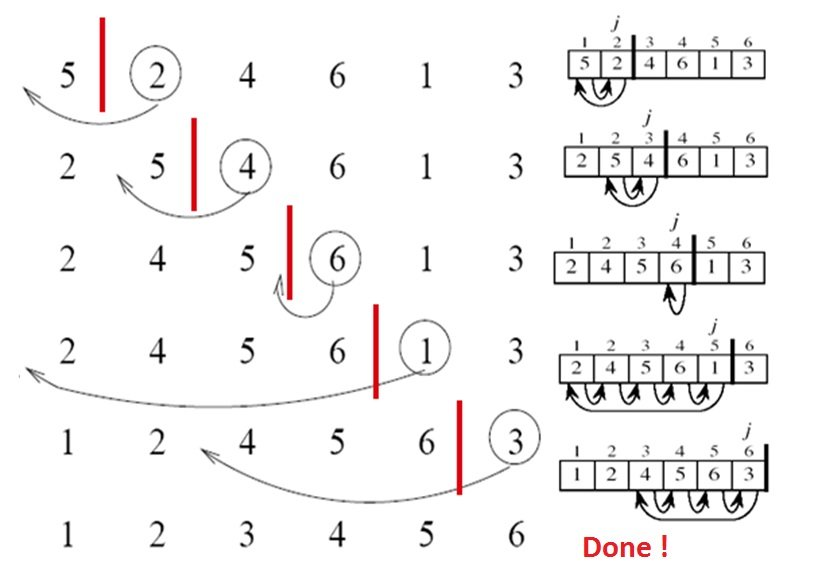## Insertion Sort

Insertion sort is a sorting algorithm that builds a sorted list one element at a time from the unsorted list. Although it is not very efficient on large datasets, it is very efficient on small datasets. It is also quite simple to undserstand. In fact, most humans when sorting—ordering a deck of cards, for example—use a method that is similar to insertion sort. Every repetition of insertion sort removes an element from the input data, inserting it into the correct position in the already-sorted list, until no input elements remain. Sorting is typically done in-place.## Insertion Sort Complexity

Insertion sort is noted for its simplicity, and its efficiency with small datasets. But Insertion sort has O(n2) complexity, making it inefficient on large datasets. The most simple worst-case scenario is an array sorted in reverse order. The set of all worst case inputs consists of all arrays where each element is the smallest or second-smallest of the elements before it. In these cases every iteration of the inner loop will scan and shift the entire sorted subsection of the array before inserting the next element. This gives insertion sort a quadratic running time (i.e., O(n2)). The average case is also quadratic, which makes insertion sort inefficient for sorting large datasets. The best case input is an array that is already sorted. In this case insertion sort has a linear running time (O(n)).

## Insertion Sort Algorithm

Below is an example of Insertion Sort written in Java..

```package miftyisbored;

/**
*
* @author m_yusuf
*	This is a small class that implements Insertion Sort
*/
public class MiftyInsertionSort {
/**
* This main method is used to illustrate how to use the Insertion Sort Algorithm
**/
public static void main(String[ ] args)
{
final String BLANK_SPACE = "  "; // Just because I am lazy :)
int[ ] data = { 10000, 1002, 83, 12, 58, 72, 66, 65, 75648, 90, 23, 37, 41, 66, 0, 3100, 55555, 1, 67 };

// Print the array before sorting:
System.out.println("Welcome to Mifty's Insertion Sorter");
System.out.println("Here is the original unsorted list:");
for (int i = 0; i < data.length; i++)
System.out.print(data[i] + BLANK_SPACE);
System.out.println( );

// Sort the numbers, and print the results
insertionSort(data);
System.out.println("All numbers are sorted now!");
System.out.println("Here is the ordered list:");
for (int i = 0; i < data.length; i++)
System.out.print(data[i] + BLANK_SPACE);
System.out.println( );
}

/**
* This function sorts an array of integers from smallest to largest using selection sort
* @param: data - the array to be sorted
*/
public static void insertionSort(int[ ] data){

int length = data.length;  // get the size of elements
int tempValue; // Used during the swapping of two array values

for (int i = 1; i < length; i++) {
for(int j = i ; j > 0 ; j--){
if(data[j] < data[j-1]){
tempValue = data[j];
data[j] = data[j-1];
data[j-1] = tempValue;
}
}
}
}
}

```

The following is the output of the application

```Welcome to Mifty's Insertion Sorter
Here is the original unsorted list:
10000  1002  83  12  58  72  66  65  75648  90  23  37  41  66  0  3100  55555  1  67
All numbers are sorted now!
Here is the ordered list:
0  1  12  23  37  41  58  65  66  66  67  72  83  90  1002  3100  10000  55555  75648
```

Mifty Yusuf is a Montreal-based software developer who enjoys playing with new web technologies as well as comic books and illustrations. He beleives that, no matter what the question is, the answer is always Batman!